In this game you there will be a equation and you will need to calculate the answer to the equation and then whack the object with the answer on it. Note that if you take too long to calcualte, then the object with the answer may return to the hole and you will need to spend time to wait for the object to appear again. The faster you whack the correct object, the higher your score. If you whack the wrong object, scores will be deducted. After you have finished one question, another question will appear and the questions will be harder and harder. There are 10 questions in this game, finish as fast and as accurate as possible to score high marks.

## Related software (5)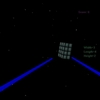## Galactic Geometry 3D

Calculate volume and surface area to blast away rocks and save your space ship! Galactic Geometry is an engaging 3D environment for learning about geometric figures and their equat ...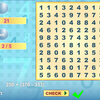## Math Search

Solve the equations and look for the answers on the grid.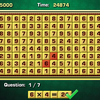## Arithmetic Game

Complete the equations in the interesting arithmetic test!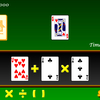## Make 24

Use the given cards to form a valid equation within 60 seconds!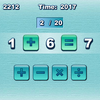## Quick Math

How quickly can you complete the equations with arithmetic symbols?